# My father

My father has a big farm. 6/8 of it were planted with mango trees, 1/2 of the remainder are guava trees and the rest 10 trees are santol trees. What is the number of all trees?

n =  80

### Step-by-step explanation:

6/8 n + 1/2 • (n - 6/8n) + 10 = n

6/8•n + 1/2 • (n - 6/8•n) + 10 = n

n = 80

Our simple equation calculator calculates it.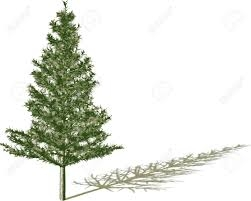Did you find an error or inaccuracy? Feel free to write us. Thank you!

Showing 1 comment:Charlie
I have written my own equation like this: Let x is the total number of trees, then, the equation will be like this:

6x/8+2x/16+10=x
12x/16+2x/16+160/16=x
14x/16+160/16=x
16x=14x+160
16x-14x=160
2x=160
2x/2=160/2
x=80Tips to related online calculators
Need help to calculate sum, simplify or multiply fractions? Try our fraction calculator.
Do you have a linear equation or system of equations and looking for its solution? Or do you have a quadratic equation?

## Related math problems and questions:

• TreesFrom the total number of trees in the orchard, there are two-fifths pearls and apples are three eighty. The rest of the trees are 9 ceremonial. How many trees are in the set?
• Discount saleAfter the discount, the computer costs 9600, - CZK. How much did it cost when the price was reduced: a) by half b) by a third c) by one fifth and then by 160 CZK
• DivisibilityDetermine the smallest integer which divided 11 gives remainder 4 when divided 15 gives remainder 10 and when divided by 19 gives remainder 16.
• Promile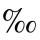Calculate the 4.6 ‰ of 199.
• Trees3/5 trees are apples, cherries are 1/3. 5 trees are pear. How many is the total number of trees?
• SixteenSixteen minus the quotient of 12 and what number is thirteen?
• DivisionWhich number in division 16 give 12 and the rest 3?
• There 11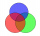There are 50 pupil in the class. Out of of this number,1/10 speak French only and 4/5 of the 10 remainder speak both French and English . If the rest speak English only find the number of students who speak only English?
• Big number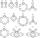The hat is the remainder when dividing number 10 to 47 - 111 by number 9?
• Birthday party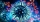For her youngest son's birthday party, the mother bought 6 3/4 kg of hotdog and 5 1/3 dozens bread rolls. Hotdogs cost 160 per kilogram, and a dozen bread rolls cost 25. How much did she spend in all?
• Unknown numberI think the number: Its half is 16 bigger than his quarter. I think the number N . ..
• VariableFind variable P: PP plus P x P plus P = 160
• Proportion 3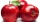For every 8 mango trees in the orchard, there are 4-star apple trees. If there are 1320 trees, how many trees of each kind are there?
• Car rent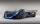Rental cars are paid a fixed daily fee plus 16 cents for each kilometer traveled. Samuel want to rent a car for a day and worked out that will pay 175 euros. When paying he got a 20% discount on the daily fee, the cost per kilometer is unchanged. Samuel t
• An average a day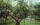Forest workers planted 96 trees on Monday, 120 trees on Tuesday, and 61 trees on Wednesdays. How many trees did she plant in the last day of Thursday, if she planted an average of 105 trees a day?
• Father and son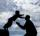Father is 44 years old, his son 16 years. Determine how many years ago was the father five times older than the son.
• The same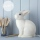The same number of rabbits and hens are running on the farm. 468 feet run around the yard. How many rabbits and hens do they have on the farm?